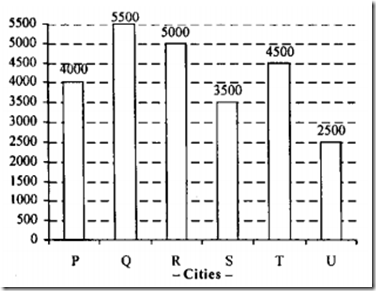## Tuesday, 22 April 2014

Directions (Q 1-5) : Study the following table carefully and Answer the Questions given below
Percentage Distribution of Students in various discipline in five different colleges
 Discipline → Colleges ↓ Arts Commerce Science Total number of students A 25 35 40 17500 B 15 45 40 25000 C 15 30 55 35300 D 28 48 24 23000 E 29 30 41 32400
1. What is the average number of students from the  discipline of Commerce from all the colleges together?
1)9745   2)9735   3)9720
4)9750 5) None of these

2. Which college has the least number of students from  the discipline of Science ?
1)A 2)C 3)E
4) D 5) None of these

3. What is the difference between the total number of  students from the discipline of Arts from all the colleges  together and the total number of students from the  discipline of Science from all the colleges together?
1)22874 2)23863 3)22963
4)23874 5) None of these

4. What is the ratio of the number of students from the  disciplines of Arts and Commerce together from college  A to the number of students from the same disciplines
from college B?
1)6:11 2)7:9 3)2:3
4)7:10 5) None of these

5. The number of students from the discipline of Arts from  college D is approximately what percentage of the  number of students from the discipline of Commerce from
the same college?
1)61 2)58 3)53
4)63 5)49
Directions (Q. 6-10) Study the graph carefully to  answer the following questions.
Number of people contributing towards helping  Tsunami victims from different cities6. The number of people contributing from cities Q and U  together forms what per cent of the total number of  people contributing from all the given cities?
1)30 2)36 3)32
4)38 5) None of these
7. Approximately by what per cent the number of people  contributing from City T is more in comparision to the  number of people contributing from City S?
1)38 2)18 3)22
4)29 5)34
8. What is the ratio of the number of people contributing  from City P to that from City R?
1)5:4 2)4:7 3)7:4
4)5:9
5) None of these

9. The number of people contributing from how many cities  forms less than 18% of the total number of people  contributing from all the cities together?
1)3 2)4 3)2
4) 1 5) None of these

10. How many more people from City S would have made
the ratio 11 : 9 for the number of people contributing
from City S to that from City T?
1)1500 2)2000 3)5500
4)3500 5) None of these
 Q.no 1 2 3 4 5 6 7 8 9 10 Answer 1 4 5 4 2 3 4 5 1 2
All The Best
Also Read : SBI PO Descriptive Model Questions
If You Have Questions Please Make A Comment Below

1.answer to first question is 9780 and should be NONE of THESE i.e 5 option in the choice

1.please Check in Question Under for clarification

2.can you please solve the question no.3 i get answer as 3 option 22963

1.to calculate faster, instaed of Finding all the students of arts and science Stream first and then subtracting , lets assume that in each college science students are more and keep subtracting the number of arts students college wise
Difference = (40% of 17500-25% of 17500)+( 40% of 25000-15% of 25000)+ (55% of 35300-15% of 35300)+(24% of 23000-28% of 23000)+(41% of 32400-29% of 32400)

= (15x175)+(25x250)+(40x353)+(-4x 230)+(12x324)
= 2625+ 6250+14120-920+3888

total :25963

2.Or can we add all % of science & then add arts ,,then find numb of students ? by subtracting?? plz tell i tried bt got 117216 as ans :/

3.pls help me 2 solve questn 4,7 nd 10

4.Please verify the question the percentage she be 35 for college A commerce. else all answer tat u provided will be wrong. if you cross check total percentage for college A is 101(25+36+40=101) which is wrong so the value she be 35 instead of 36.

1.5.can you please solve 7th question and 10th question.

1.7th : (4500-3500/3500)x100 = 28.57% so Approximately 29

2.10 : S:T = 11:9= 5500:4500

WHICH MEANS THERE SHOULD BE 5500-3500= 2000 more People from City S

6.soln. of ques 3

(40-25)*175= 2625
(40-15)*250= 6250
(55-15)*353=14120
(24-28)*230= - 920
(41-29)*324= 3888

(2625+6250+14120-920+3888 = 25963)
hence option (e) is correct